#### 1.  Find the gradient of the graph y = 5

(a) From answer 1 of Straight Line Graphs is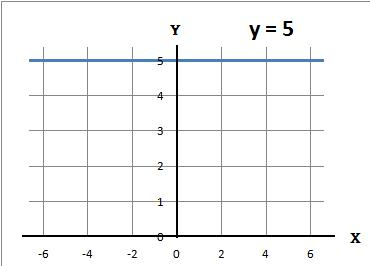(b) Work out the gradient. From the graph every time x increases by 1, y increases by 0 so

#### 2.  Find the gradient of the graph y = x − 3

(a) From answer 2 of Straight Line Graphs is(b) Work out the gradient. From the graph every time x increases by 1, y increases by 1 so

#### 3.  Find the gradient of the graph y = −x

(a) From answer 3 of Straight Line Graphs is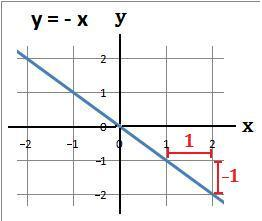(b) Work out the gradient. From the graph every time x increases by 1, y decreases by 1 so

#### 4.  Find the gradient of the graph y = −2 − x

(a) From answer 4 of Straight Line Graphs is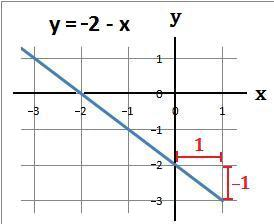(b) Work out the gradient. From the graph every time x increases by 1, y decreases by 1 so

#### 5.  Find the gradient of the graph y = 3x + 4

(a) From answer 5 of Straight Line Graphs is(b) Work out the gradient. From the graph every time x increases by 2, y increases by 6 so

#### 6.  Find the gradient of the graph y = 3 − 2x

(a) From answer 6 of Straight Line Graphs is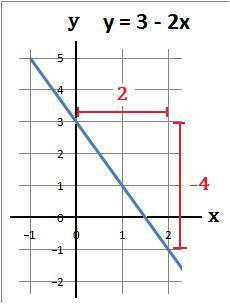(b) Work out the gradient. From the graph every time x increases by 2, y decreases by 4 so

#### 7.  Find the gradient of the graph 2y − x = 5

(a) From answer 7 of Straight Line Graphs is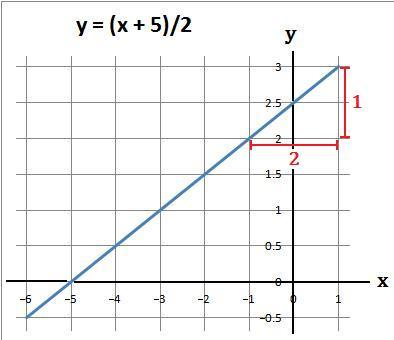(b) Work out the gradient. From the graph every time x increases by 2, y increases by 1 soback to: